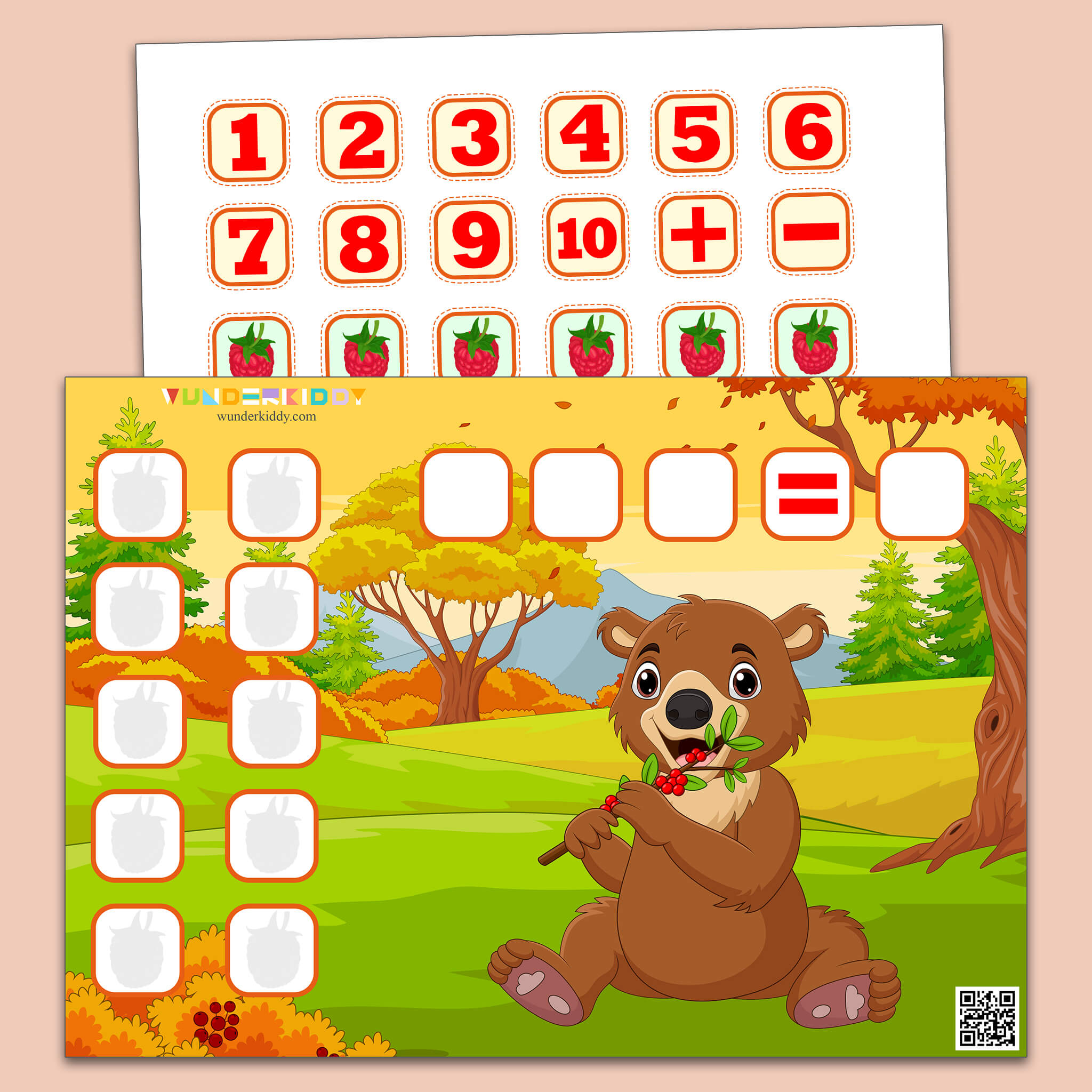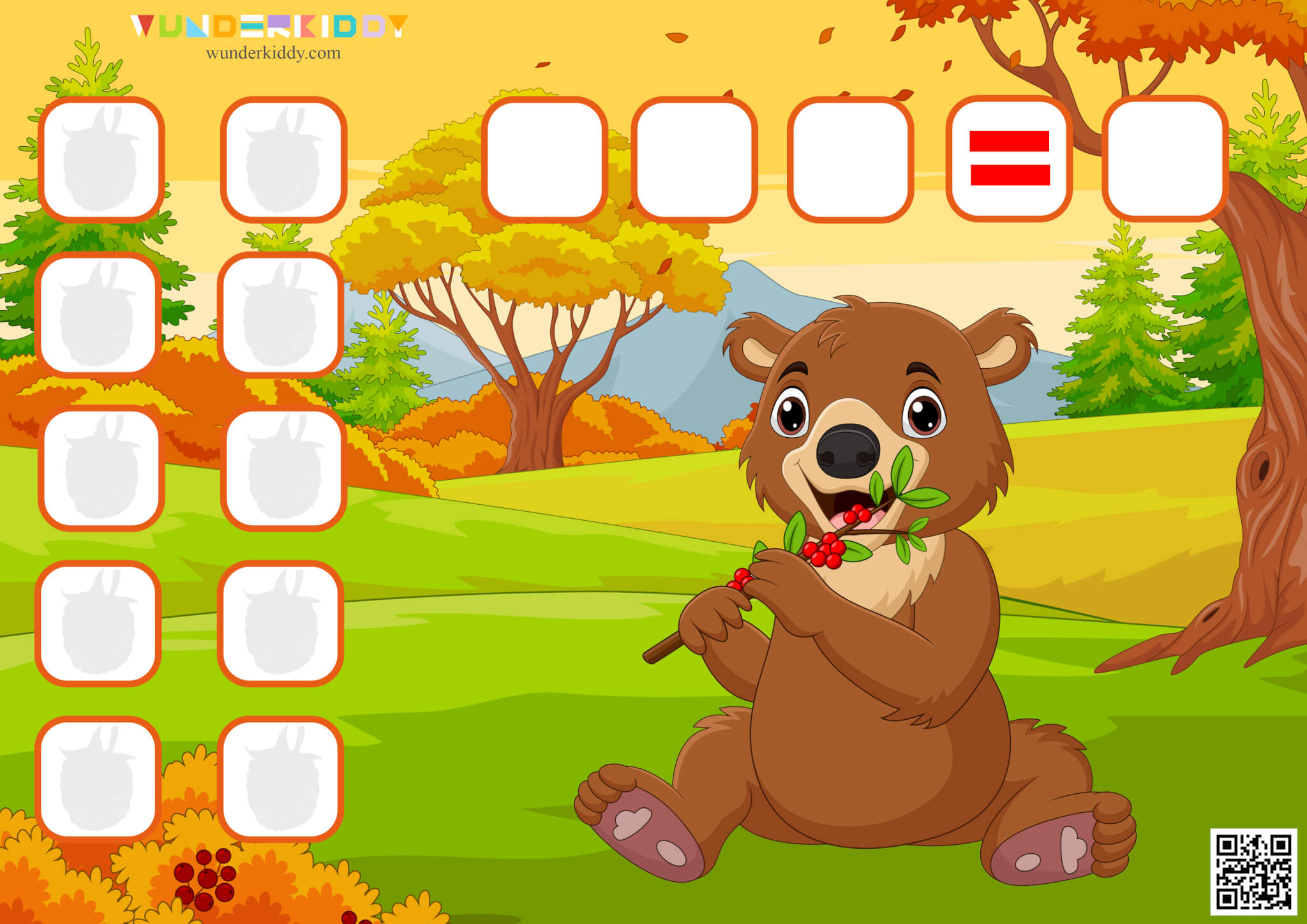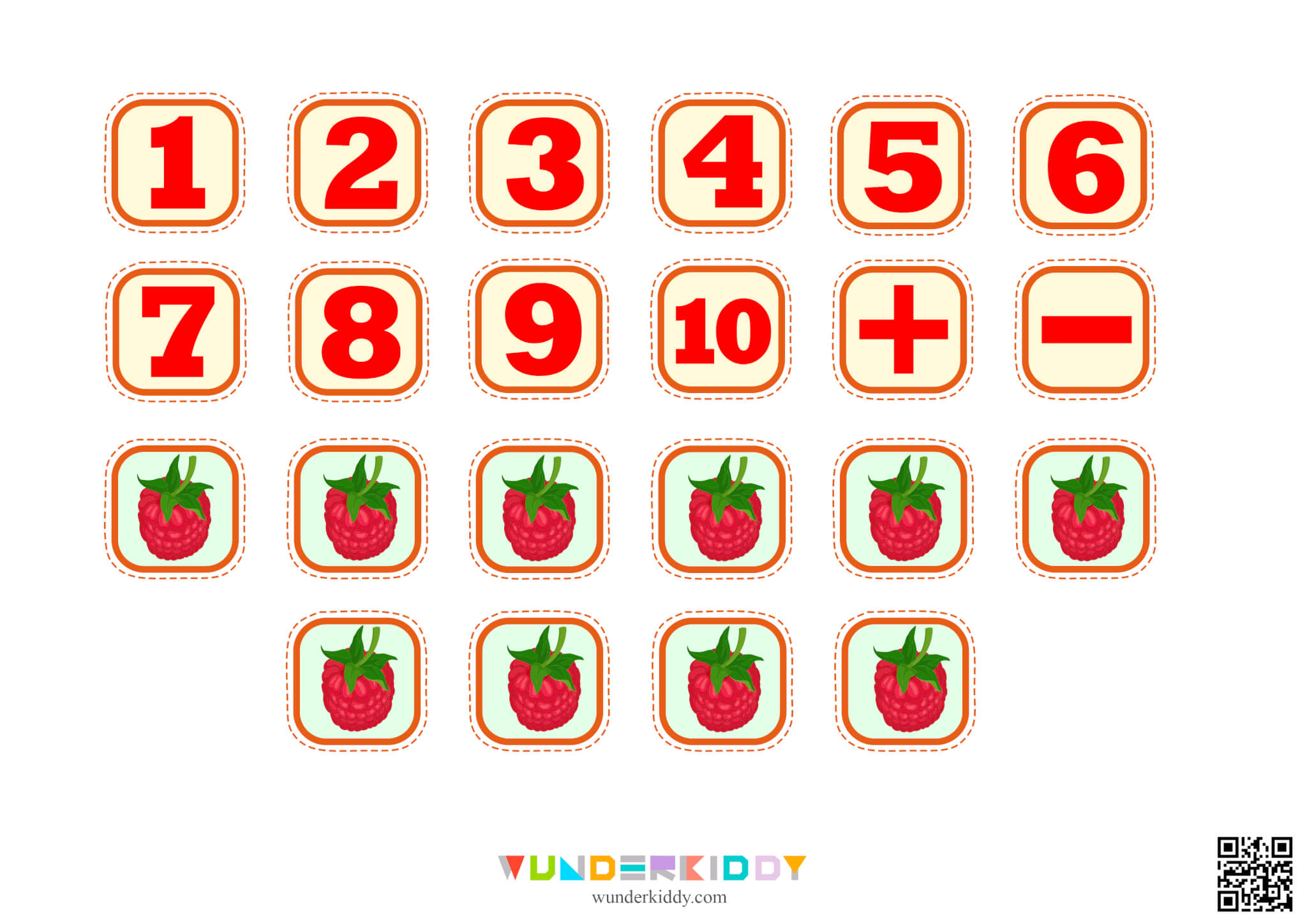GET IT X
Free

# Addition and Subtraction Practice The Bear and RaspberryThe «Bear and Raspberries» free printable simple math worksheet for children ages 5 and up will teach your child how to calculate the math operations of addition and subtraction within 10 using visual examples. Print out the math activity to practice math thinking and fine motor skills.

The task is to count how many berries the bear ate and how many are left. Explain to the child what addition and subtraction of numbers are, how they are performed, show the symbols that represent these operations.

The top part of the figure shows a template for a math example. Create a math example, put the numbers and the «+» or «-» signs in it. Use cards with berries to teach your child to perform these actions. For example, your baby needs to calculate 2+1. Ask him or her to count and put two berries in the corresponding boxes, then add one more. Then count the total number of the berries and put the digit of the number card in the answer box.

Cristina Mihaela
+3
I like this worksheet very much
Aveen Jamo
+3
I like it
Nathalia Cavalcante
+1